# 2.4 Case Study: Percolation

We conclude our study of functions and modules by considering a case study of developing a program to solve an interesting scientific problem: a Monte Carlo simulation to study a natural model known as percolation.

## Percolation

We model the system as an n-by-n grid of sites. Each site is either blocked or open; open sites are initially empty. A full site is an open site that can be connected to an open site in the top row via a chain of neighboring (left, right, up, down) open sites. If there is a full site in the bottom row, then we say that the system percolates. If sites are independently set to be open with vacancy probability p, what is the probability that the system percolates? No mathematical solution to this problem has yet been derived. Our task is to compose computer programs to help study the problem.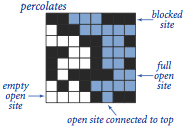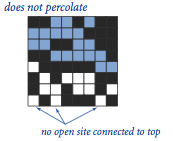## Scaffolding

Our first step is to pick a representation of the data. We use one boolean matrix to represent which sites are open and another boolean matrix to represent which sites are full. We will design a `flow()` function that takes as an argument a two-dimensional boolean array `isOpen` that specifies which sites are open and returns another two-dimensional boolean array `isFull` that specifies which sites are full. We will also include a `percolates()` function that checks whether the array returned by `flow()` has any full sites on the bottom.

## Vertical Percolation

Given a boolean matrix that represents the open sites, how do we figure out whether it represents a system that percolates? For the moment, we will consider a much simpler version of the problem that we call vertical percolation. The simplification is to restrict attention to vertical connection paths.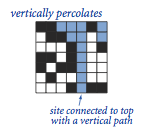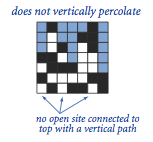Determining the sites that are filled by some path that is connected vertically to the top is a simple calculation.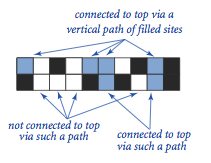The program percolationv.py is a solution to the vertical-only percolation problem. Try running it with its standard input redirected to test5.txt or text8.txt

## Monte Carlo Simulation

We want our code to work properly for any boolean matrix. Moreover, the scientific question of interest involves random boolean matrices. To this end, we compose a function `random()` that takes two arguments `n` and `p` and generates a random `n`-by-`n` boolean array in which the probability that each element is `True` is `p`. That function is defined in percolationio.py.

## Data Visualization

We can work with bigger problem instances if we use `stddraw` for output. Accordingly we develop a function `draw()` to visualize the contsnts of a boolean matrix. That function, along with the aforementioned `random()` function and a test `main()` function, is defined in percolationio.py.

 `% python percolationio.py 10 0.8`Program visualizev.py is a test client of percolationv.py that generates random boolean matrices by calling `percolationio.random()` and plots them to standard draw by calling `percolationio.draw()`. It generates output such as this:

 `% python visualizev.py 20 0.95 1` `% python visualizev.py 20 0.95 1`## Estimating Probabilities

The next step in our program development process is to compose code to estimate the probability that a random system (of size n with site vacancy probability p) percolates. We refer to this quantity as the percolation probability. To estimate its value, we simply run a number of experiments. Program estimatev.py encapsulates this computation in a function `evaluate()`.

## Recursive Solution for Percolation

How do we test whether a system percolates in the general case when any path starting at the top and ending at the bottom (not just a vertical one) will do the job? Remarkably, we can solve this problem with a compact program, based on a classic recursive scheme known as depth-first search. Program percolation.py contains an implementation of `flow()` that computes the flow array. Try running it with its standard input redirected to test5.txt and then text8.txt

The programs visualize.py and estimate.py are identical to visualizev.py and estimatev.py respectively, except that they are clients of percolation.py instead of percolationv.py. The visualize.py program generates output such as this: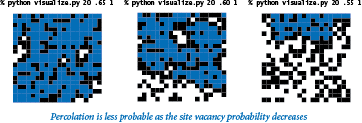To gain more insight into percolation, the next step in program development is to compose a program that plots the percolation probability as a function of the site vacancy probability p for a given value of n. Immediately, we are faced with numerous decisions. For how many values of p should we compute an estimate of the percolation probability? Which values of p should we choose? Program percplot.py implements a recursive appraoch that produces a good-looking curve at relatively low cost.

 `% python percplot.py 20` `% python percplot.py 100`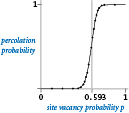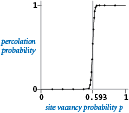The curves support the hypothesis that there is a threshold value (about .593): if p is greater than the threshold, then the system almost certainly percolates; if p is less than the threshold, then the system almost certainly does not percolate. As n increases, the curve approaches a step function that changes value from 0 to 1 at the threshold. This phenomenon, known as a phase transition, is found in many physical systems.

## Lessons

• Expect bugs. Every interesting piece of code that you compose is going to have at least one or two bugs, if not many more. By running small pieces of code on small test cases that you understand, you can more easily isolate any bugs and then more easily fix them when you find them.
• Keep modules small. You can focus attention on at most a few dozen lines of code at a time, so you may as well break your code into small modules as you compose it.
•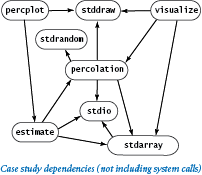• Limit interactions. In a well-designed modular program, most modules should depend on just a few others. In particular, a module that calls a large number of other modules needs to be divided into smaller pieces. Modules that are called by a large number of other modules (you should have only a few) need special attention, because if you do need to make changes in a module's API, you have to reflect those changes in all its clients.
• Develop code incrementally. You should run and debug each small module as you implement it. That way, you are never working with more than a few dozen lines of unreliable code at any given time.
• Solve an easier problem. It is typical to begin by putting together the simplest code that you can craft that solves a given problem, as we did with vertical-only percolation.
• Consider a recursive solution. Recursion is an indispensable tool in modern programming that you should learn to trust. If you are not already convinced of this fact by the simplicity and elegance of the `flow()` method in percolation.py, you might wish to try to develop a nonrecursive version.
• Build tools when appropriate. Our visualization function `draw()` and random boolean matrix generation function `random()` are certainly useful for many other applications, as is the adaptive plotting function of percplot.py. Incorporating these functions into appropriate modules would be simple.
• Reuse software when possible. Our `stdio`, `stdrandom`, and `stddraw` modules all simplified the process of developing the code in this section.

#### Q & A

Q. Editing visualize.py and estimate.py to rename every occurrence of `percolation` to `percolationv` (or whatever module we want to study) seems to be a bother. Is there a way to avoid doing so?

A. Yes. The most straightforward approach is to keep multiple files named `percolation.py` in separate subdirectories. Then copy the desired `percolation.py` file from one of the subdirectories to your working directory, thereby selecting one particular implementation. An alternative approach is to use Python's `import as` statement to define an identifier to refer to the module:

 ```import percolationv as percolation ```

Now, any call to `percolation.percolates()` uses the function defined in percolationv.py instead of the one defined in percolation.py. In this situation, changing implementations involves editing only one line of source code.

Q. That recursive `flow()` function makes me nervous. How can I better understand what it's doing?

A. Run it for small examples of your own making, instrumented with instructions to write a function-call trace. After a few runs, you will gain confidence that it always fills the sites connected to the start point.

Q. Is there a simple nonrecursive approach?

A. There are several known approaches that perform the same basic computation. We will revisit the problem at the end of the book, in Section 4.5. In the meantime, working on developing a nonrecursive implementation of `flow()` is certain to be an instructive exercise, if you are interested.

Q. The percplot.py program seems to involve a huge amount of calculation to get a simple function graph. Is there some better way?

A. The best strategy would be a mathematical proof of the threshold value, but that derivation has eluded scientists.

Q. What is the percolation threshold for different lattices?

A. percolation threshold probability p* such that if p < p* then no spanning cluster exists, and if p >= p* then one spanning cluster exists.

Q. Any relevant papers?

A. See A Fast Monte Carlo Algorithm for Site or Bond Percolation by Newman and Ziff.

#### Exercises

1. Compose a program that takes a command-line argument n and creates an n-by-n matrix with the entry in row i and column j set to `True` if `i` and `j` are relatively prime, then shows the n-by-n boolean array on the standard drawing (see the "relatively prime" exercise in Section 1.4.). Then, compose a similar program to draw the Hadamard matrix of order n (see the "Hadamard matrix" creative exercise in Section 1.4) and another program to draw the matrix with the element in row i and column j set to `True` if the coefficient of xj in (1+x)i (binomial coefficient) is odd (see the "random walkers" creative exercies in Section 1.4). You may be surprised at the pattern formed by the latter.

2. Compose a `write()` function for percolationio.py that writes 1 for blocked sites, 0 for open sites, and * for full sites.

3. Give the recursive calls for percolation.py given the following input:

 ```3 3 1 0 1 0 0 0 1 1 0 ```
4. Compose a client of percolation.py like visualize.py that does a series of experiments for command-line argument n where the site vacancy probability p increases from 0 to 1 by a given increment (also taken from the command line).

5. Compose a program `percolationd.py` that tests for directed percolation (by leaving off the last recursive call in the recursive `_flow()` function in the percolation.py program. Then use percplot.py (suitably modified) to draw a plot of the directed percolation probability as a function of the site vacancy probability.

6. Compose a client of percolation.py and `percolationd.py` that takes a site vacancy probability p from the command line and writes an estimate of the probability that a system percolates but does not percolate down. Use enough experiments to get an estimate that is accurate to three decimal places.

7. Describe the order in which the sites are marked when percolation.py is used on a system with no blocked sites. Which is the last site marked? What is the depth of the recursion?

8. Modify percolation.py to animate the flow computation, showing the sites filling one by one. Check your answer to the previous exercise.

9. Experiment with using percplot.py to plot various mathematical functions (just by replacing the call of `estimate.evaluate()` with an expression that evaluates the function). Try the function `sin(x) + cos(10*x)` to see how the plot adapts to an oscillating curve, and come up with interesting plots for three or four functions of your own choosing.

10. Modify percolation.py to animate the flow computation, showing the sites filling one by one. Check your answer to the previous exercise.

11. Modify percolation.py to compute that maximum depth of the recursion used in the flow calculation. Plot the expected value of that quantity as a function of the site vacancy probability p. How does your answer change if the order of the recursive calls is reversed?

12. Modify estimate.py to produce output like that produced by bernoulli.py (from Section 2.2). Extra credit: Use your program to validate the hypothesis that the data obeys the Gaussian (normal) distribution.

13. Modify percolationio.py, estimate.py, percolation.py, and visualize.py to handle m-by-n grids and m-by-n boolean matrices. Use an optional argument so that m defaults to n if only one of the two dimensions is specified.

#### Creative Exercises

1. Vertical percolation. Show that an n-by-n percolation system with site vacancy probability p vertically percolates with probability 1 - (1 - pn)n, and use estimate.py to validate your analysis for various values of n.

2. Rectangular percolation systems. Modify the code in this section to allow you to study percolation in rectangular systems. Compare the percolation probability plots of systems whose ratio of width to height is 2:1 with those whose ratio is 1:2.

3. Adaptive plotting. Modify percplot.py to take its control parameters (gap tolerance, error tolerance, and number of trials) from the command line. Experiment with various values of the parameters to learn their effect on the quality of the curve and the cost of computing it. Briefly describe your findings.

4. Percolation threshold. Compose a percolation.py client that uses binary search to estimate the threshold value (see the "Binary search" creative exercise in Section 2.1).

5.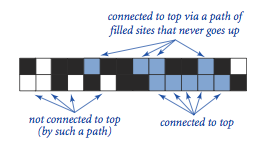Nonrecursive directed percolation. Compose a nonrecursive program that tests for directed percolation by moving from top to bottom as in our vertical percolation code. Base your solution on the following computation: if any site in a contiguous subrow of open sites in the current row is connected to some full site on the previous row, then all of the sites in the subrow become full.

6. Fast percolation test. Modify the recursive `_flow()` function in percolation.py so that it returns as soon as it finds a site on the bottom row (and fills no more sites). Hint: Use an argument `done` that is `True` if the bottom has been hit, `False` otherwise. Give a rough estimate of the performance improvement factor for this change when running percplot.py Use values of n for which the programs run at least a few seconds but not more than a few minutes. Note that the improvement is ineffective unless the first recursive call in `_flow()` is for the site below the current site.

7.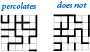Bond percolation. Compose a modular program for studying percolation under the assumption that the edges of the grid provide connectivity. That is, an edge can be either empty or full, and a system percolates if there is a path consisting of full edges that goes from top to bottom. Note: This problem has been solved analytically, so your simulations should validate the hypothesis that the percolation threshold approaches 1/2 as n gets large.

8.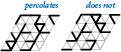Bond percolation on a triangular grid. Compose a modular program for studying bond percolation on a triangular grid, where the system is composed of 2n2 equilateral triangles packed together in an n-by-n grid of rhombus shapes and, as in the previous exercise, the edges of the grid provide the connectivity. Each interior point has six bonds; each point on the edge has four; and each corner point has two.

9. Percolation in three dimensions. Implement modules `percolation3d.py` and percolation3dio.py (for I/O and random generation) to study percolation in three-dimensional cubes, generalizing the two-dimensional case studied in this chapter. A percolation system is an n-by-n-by-n cube of sites that are unit cubes, each open with probability p and blocked with probability 1-p. Paths can connect an open cube with any open cube that shares a common face (one of six neighbors, except on the boundary). The system percolates if there exists a path connecting any open site on the bottom plane to any open site on the top plane. Use a recursive version of `_flow()` like the one in percolation.py, but with six recursive calls instead of four. Plot percolation probability versus site vacancy probability for as large a value of n as you can. Be sure to develop your solution incrementally, as emphasized throughout this section.

10. Game of life. Implement a module `life.py` that simulates Conway's game of life. Consider a boolean matrix corresponding to a system of cells that we refer to as being either live or dead. The game consists of checking and perhaps updating the value of each cell, depending on the values of its neighbors (the adjacent cells in every direction, including diagonals). Live cells remain live and dead cells remain dead, with the following exceptions:

• A dead cell with exactly three live neighbors becomes live.
• A live cell with exactly one live neighbor becomes dead.
• A live cell with more than three live neighbors becomes dead.

Test your program with a glider, a famous pattern that moves down and to the right every four generations, as shown in the diagram below. Then try two gliders that collide. Then try a random boolean matrix. This game has been heavily studied, and relates to foundations of computer science.